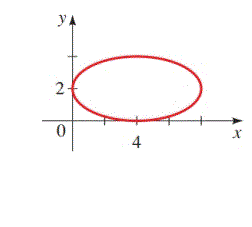Chapter 12.CR, Problem 41E### Algebra and Trigonometry (MindTap ...

4th Edition
James Stewart + 2 others
ISBN: 9781305071742

#### Solutions

Chapter
Section### Algebra and Trigonometry (MindTap ...

4th Edition
James Stewart + 2 others
ISBN: 9781305071742
Textbook Problem

# 37-42 ■ Finding the Equation of a Conic Find an equation for the conic whose graph is shownTo determine

To find:

The equation for the conic whose graph is given.

Explanation

Given:

Figure 1

Approach:

Draw the major and minor axes of the given ellipse figure. Intersection point of the major and minor axes, is the center of the ellipse and from the length of the major and minor axes, value of a and b can be found.

Calculation:

Draw the major and minor axes of the given ellipse figure as shown below,

Figure 2

From the figure, the intersection point of major and minor axes is (4,2). Since the intersection point of the major and minor axes, is the center of the ellipse. So center (h,k)=(4,2)

The graph is of horizontal ellipse, and the length of major axis is 2a=8 and the length of minor axis is 2b=4. So a=4 and b=2

### Still sussing out bartleby?

Check out a sample textbook solution.

See a sample solution

#### The Solution to Your Study Problems

Bartleby provides explanations to thousands of textbook problems written by our experts, many with advanced degrees!

Get Started